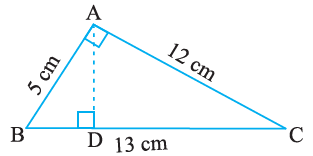# ΔABC is right angled at A (Figure). AD is perpendicular to BC. If AB = 5 cm, BC = 13 cm and AC = 12 cm, Find the area of ΔABC. Also find the length of AD.Solution

From the given figure we notet hat

AB = 5 cm

BC = 13 cm

AC = 12 cm

We know that,

Area of the ΔABC = ½ × base × height

= ½ × AB × AC

= ½ × 5 × 12

= 1 × 5 × 6

= 30 cm2

Also,

Area of ΔABC = ½ × base × height

30 = ½ × AD × BC

30 = ½ × AD × 13(0)(0)